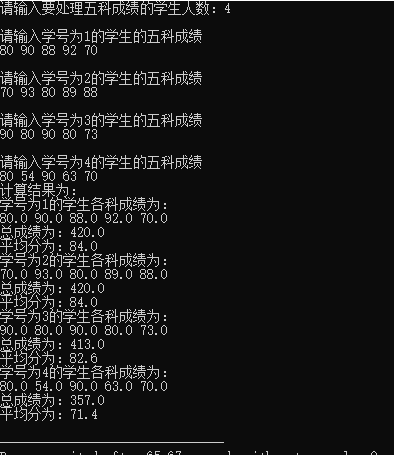# C语言练习四：指针与多维数组的相关练习

## 2、相关知识

c语言在处理数组时都是转化为同类型指针来访问的。例如int a数组，在函数内部，无论是使用下标法a[i]还是指针法*(a+i)，编译器都是将其转化为"星号(a+i)“来处理的。

## 4、程序代码

//指向数组的指针作为函数参数的运用示例
#include<stdio.h>
int main()
{
void count(float (*p)[],int);                                //声明指向数组指针处理函数
int i,j,m;                                                    //定义相关变量
printf("请输入要处理五科成绩的学生人数：");
scanf("%d",&m);                                          //存入要计算成绩的学生人数
float a[m];                                   //定义存储成绩的二维浮点型数组，行+1表学号，列表各科成绩
for(i=0;i<m;i++)
{
printf("\n请输入学号为%d的学生的五科成绩\n",i+1);
for(j=0;j<5;j++)
{
scanf("%f",&a[i][j]);     //把成绩存入二维数组 注意输入输出格式一定要与定义的类型相对应，因为浮点型与整型存储方式是不同的。
}
}
float (*p)=a;                               //定义指向数组的指针变量p并由二维数组的首地址赋初值
printf("计算结果为：\n");
count(a,m);
return 0;
}
void count(float (*p),int n)                             //定义count函数
{
int i,j;                                            //定义循环变量
for(i=0;i<n;i++)
{
float sum=0;                                 //定义累加变量并赋初值为0
printf("学号为%d的学生各科成绩为：\n",i+1);
for(j=0;j<5;j++)
{
printf("%.1f ",*(*(p+i)+j));       //再次输出成绩好对比计算结果是否正确
sum+=*(*(p+i)+j);
}
printf("\n总成绩为：%.1f\n",sum);
printf("平均分为：%.1f\n",sum/5);
}
}


## 5、运行示例©️2019 CSDN 皮肤主题: 游动-白 设计师: 上身试试